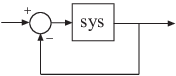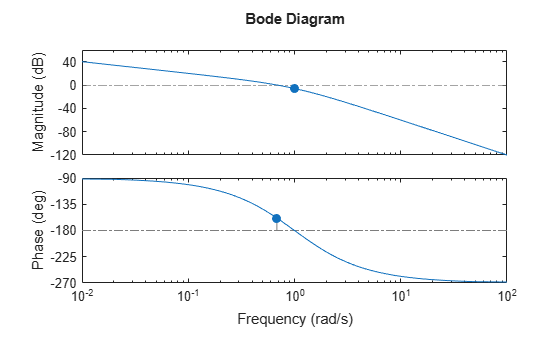Documentation

margin

Gain margin, phase margin, and crossover frequencies

Description

example

margin(sys) plots the Bode response of sys on the screen and indicates the gain and phase margins on the plot. Gain margins are expressed in dB on the plot.Solid vertical lines mark the gain margin and phase margin. The dashed vertical lines indicate the locations of Wcp, the frequency where the phase margin is measured, and Wcg, the frequency where the gain margin is measured. The plot title includes the magnitude and location of the gain and phase margin.

Gm and Pm of a system indicate the relative stability of the closed-loop system formed by applying unit negative feedback to sys, as shown in the following figure.Gm is the amount of gain variance required to make the loop gain unity at the frequency Wcg where the phase angle is –180° (modulo 360°). In other words, the gain margin is 1/g if g is the gain at the –180° phase frequency. Similarly, the phase margin is the difference between the phase of the response and –180° when the loop gain is 1.0.

The frequency Wcp at which the magnitude is 1.0 is called the unity-gain frequency or gain crossover frequency. Usually, gain margins of three or more combined with phase margins between 30° and 60° result in reasonable tradeoffs between bandwidth and stability.

example

[Gm,Pm,Wcg,Wcp] = margin(sys) returns the gain margin Gm in absolute units, the phase margin Pm, and the corresponding frequencies Wcg and Wcp, of sys. Wcg is the frequency where the gain margin is measured, which is a –180° phase crossing frequency. Wcp is the frequency where the phase margin is measured, which is a 0-dB gain crossing frequency. These frequencies are expressed in radians/TimeUnit, where TimeUnit is the unit specified in the TimeUnit property of sys. When sys has several crossovers, margin returns the smallest gain and phase margins and corresponding frequencies.

example

[Gm,Pm,Wcg,Wcp] = margin(mag,phase,w) derives the gain and phase margins from frequency response data. Provide the gain data mag in absolute units, and phase data phase in degrees. You can provide the frequency vector w in any units and margin returns Wcg and Wcp in the same units.

example

[Gm,Pm] = margin(sys,J1,...,JN) returns the gain margin Gm and phase margin Pm of the entries in model array sys with subscripts (J1,...,JN).

Examples

collapse all

For this example, create a continuous transfer function.

sys = tf(1,[1 2 1 0])
sys =

1
---------------
s^3 + 2 s^2 + s

Continuous-time transfer function.

Display the gain and phase margins graphically.

margin(sys)The gain margin (6.02 dB) and phase margin (21.4 deg), displayed in the title, are marked with solid vertical lines. The dashed vertical lines indicate the locations of Wcg, the frequency where the gain margin is measured, and Wcp, the frequency where the phase margin is measured.

For this example, create a discrete-time transfer function.

sys = tf([0.04798 0.0464],[1 -1.81 0.9048],0.1)
sys =

0.04798 z + 0.0464
---------------------
z^2 - 1.81 z + 0.9048

Sample time: 0.1 seconds
Discrete-time transfer function.

Compute the gain margin, phase margin and frequencies.

[Gm,Pm,Wcg,Wcp] = margin(sys)
Gm = 2.0517
Pm = 13.5711
Wcg = 5.4374
Wcp = 4.3544

The results indicate that a gain variation of over 2.05 dB at the gain crossover frequency of 5.43 rad/s would cause the system to be unstable. Similarly a phase variation of over 13.57 degrees at the phase crossover frequency of 4.35 rad/s will cause the system to lose stability.

For this example, load the frequency response data of an open loop system, consisting of magnitudes (m) and phase values (p) measured at the frequencies in w.

Compute the gain and phase margins.

[Gm,Pm,Wcg,Wcp] = margin(m,p,w)
Gm = 0.6249
Pm = 48.9853
Wcg = 1.2732
Wcp = 1.5197

For this example, load invertedPendulumArray.mat, which contains a 3-by-3 array of inverted pendulum models. The mass of the pendulum varies as you move from model to model along a single column of sys, and the length of the pendulum varies as you move along a single row. The mass values used are 100g, 200g and 300g, and the pendulum lengths used are 3m, 2m and 1m respectively.

$\begin{array}{cccc}& Column\phantom{\rule{0.5em}{0ex}}1& Column\phantom{\rule{0.5em}{0ex}}2& Column\phantom{\rule{0.5em}{0ex}}3\\ Row\phantom{\rule{0.5em}{0ex}}1& 100g,3m& 100g,2m& 100g,1m\\ Row\phantom{\rule{0.5em}{0ex}}2& 200g,3m& 200g,2m& 200g,1m\\ Row\phantom{\rule{0.5em}{0ex}}3& 300g,3m& 300g,2m& 300g,1m\end{array}$

size(sys)
3x3 array of transfer functions.
Each model has 1 outputs and 1 inputs.

Find gain and phase margin for all models in the array.

[Gm,Pm] = margin(sys)
Gm = 3×3

0.9800    0.9800    0.9800
0.9800    0.9800    0.9800
0.9800    0.9800    0.9800

Pm = 3×3

-11.3565  -11.3898  -11.4228
-11.3842  -11.4088  -11.4333
-11.4020  -11.4208  -11.4396

margin returns two arrays, Gm and Pm, in which each entry is the gain and phase margin values of the corresponding entry in sys. For instance, the gain and phase margin of the model with 100g pendulum weight and 2m length is Gm(1,2) and Pm(1,2), respectively.

Input Arguments

collapse all

Dynamic system, specified as a SISO dynamic system model, or an array of SISO dynamic system models. Dynamic systems that you can use include continuous-time or discrete-time numeric LTI models such as tf, zpk, or ss models.

If sys is a generalized state-space model genss or an uncertain state-space model uss, margin returns the gain margin and phase margin of the current or nominal value of sys. If sys is an array of models, margin returns the Gm and Pm of the model corresponding to its subscript J1,...,JN in sys. For more information on model arrays, see Model Arrays.

Indices of models in array whose gain and phase margins you want to extract, specified as positive integers. You can provide as many indices as there are array dimensions in sys. For example, if sys is a 4-by-5 array of dynamic system models, the following command extracts Gm and Pm for entry (2,3) in the array.

[Gm,Pm] = margin(sys,2,3);

Magnitude of the system response in absolute units, specified as a 3-D array. Since margin only accepts SISO systems, mag is a 1-by-1-by-N array, where N is the number of frequency points. For an example, see Obtain Magnitude and Phase Data.

Phase of the system response in degrees, specified as a 3-D array. Since margin only accepts SISO systems, phase is a 1-by-1-by-N array, where N is the number of frequency points. For an example, see Obtain Magnitude and Phase Data.

Frequencies at which the magnitude and phase values of system response are obtained, specified as a column vector.

Output Arguments

collapse all

Gain margin, returned as a scalar or an array. If sys is:

• A single model, then Gm is returned as a scalar.

• A model array, then Gm is an array of gain margins of each model in sys.

Gm is the amount of gain variance required to make the loop gain unity at the frequency Wcg where the phase angle is –180° (modulo 360°). In other words, the gain margin is 1/g if g is the gain at the –180° phase frequency. Negative gain margins indicate that stability is lost by decreasing the gain, while positive gain margins indicate that stability is lost by increasing the gain

The gain margin Gm is computed in absolute units. You can compute the gain margin in dB by,

Gm_dB = 20*log10(Gm)

Phase margin, returned as a scalar or an array. If sys is:

• A single model, then Pm is returned as a scalar.

• A model array, then Pm is an array of phase margins of each model in sys.

The phase margin is the difference between the phase of the response and –180° when the loop gain is 1.0.

The phase margin Pm is expressed in degrees.

Gain crossover frequency, returned as a scalar. Wcg is the frequency where the gain margin is measured, which is a –180° phase crossing frequency.

Wcg is expressed in radians/TimeUnit, where TimeUnit is the unit specified in the TimeUnit property of sys.

Phase crossover frequency, returned as a scalar. Wcp is the frequency where the phase margin is measured, which is a 0-dB gain crossing frequency.

Wcp is expressed in radians/TimeUnit, where TimeUnit is the unit specified in the TimeUnit property of sys.

Tips

• When you use margin(mag,phase,w), margin relies on interpolation to approximate the margins, which generally produce less accurate results. For example, if there is no 0-dB crossing within the w range, margin returns a phase margin of Inf. Therefore, if you have an analytical model sys, using [Gm,Pm,Wcg,Wcp] = margin(sys) is a more robust way to obtain the margins.